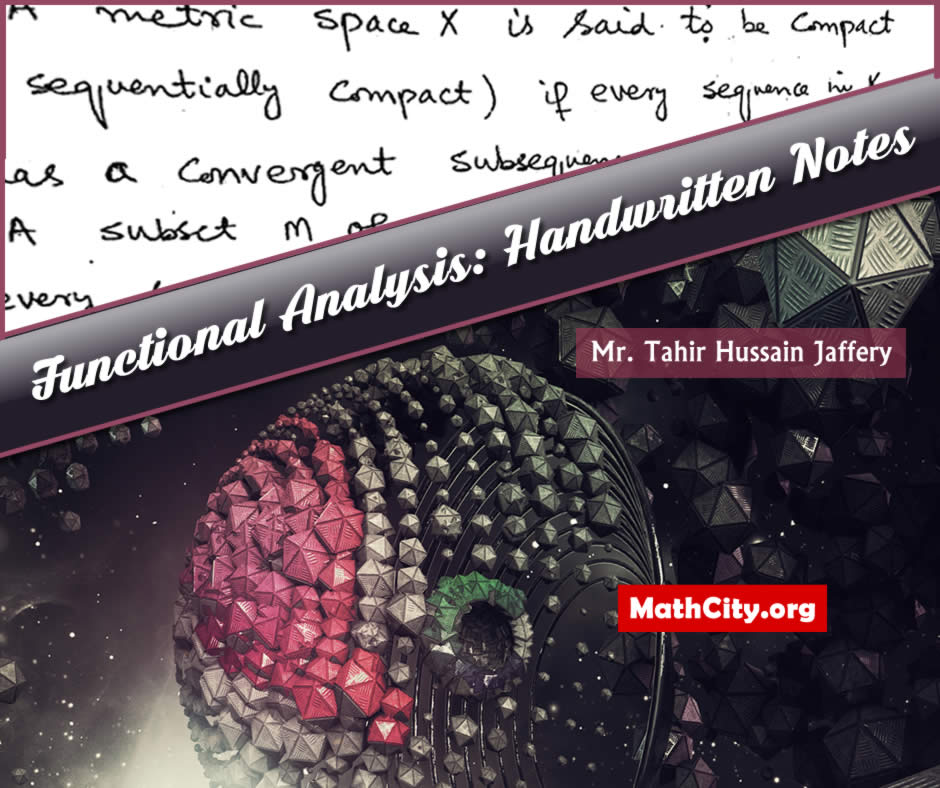# Functional Analysis by Mr. Tahir Hussain JafferyFunctional analysis is a branch of mathematics concerned with vector space theory and linear algebra. It requires looking into the relationships between various roles, objects, incidents, actions, and results. The term “functional” comes from the calculus of variations and describes a function whose argument is a function. Numerous disciplines, such as approximation theory, calculus of variations, numerical analysis, and differential and integral equations, can benefit from its use.

Handwritten notes of Functional Analysis by Mr. Tahir Hussain Jaffery of University of Peshawar. These notes are provided by Mr. Muhammad Marwan, we are very thankful to him for providing these notes. The five chapters are comprised in these notes. Each chapter is nicely written and includes both fundamental and sophisticated ideas relevant to functional analysis. Many examples are also provided to illustrate the concepts linked to functional analysis. There have been many theorems proven, particularly in the fourth chapter of these notes.

• Name: Functional Analysis: Handwritten Notes
• Written by : Mr. Tahir Hussain Jaffery
• Provided by: Mr. Muhammad Marwan
• Pages: |129 pages
• Format: Scanned PDF
• Size: 2.77 MB
• Category: Analysis
• Norm
• Examples of normed
• Normed linear space
• Bounded linear operator
• Continuous linear operator
• Norm of operator
• Isomorphism
• Isometric isomorphism
• Topological isomorphism
• Equivalent norm
• Compact space
• Holder inequality
• Minkwoski's inequality
• Complete space
• Banach space
• Convergent series in spaces
• Absolutely convergent (or absolutely summable)
• Dual space
• Algebraic dual or conjugate space
• Quotient spaces
• Equivalent module $M$
• Equivalence class
• Norm of quotient space
• Interior of a set
• Nowhere dense (or rare)
• Finite category (or meager)
• Baire's category theorem
• The principal of uniform boundedness or Banach-Steinhaus theorem
• Sublinear functional
• Semi-norm
• Extensions, restriction
• Hahn-Banach theorem (real version)
• Hahn-Banach theorem (complex version)
• Hahn-Banach theorem for non-linear spaces
• The open mapping theorem
• norm linear spaces
• Graph of T, Set in the norm linear space
• The closed graph theorem
• Inner product on complex linear space
• Consequences of the definition of inner product spaces
• Examples of inner product spaces
• Schwarz's inequality or Cauchy-Bunyakoski's inequality
• Polarization identity
• Parallelogram law for inner product spaces
• Continuous or jointly continuous
• Orthogonal and orthonormal sets
• Pythagorean thoerem
• Generalization of Pythagorean thoerem
• Orthonormal sets
• Complete orthonormal sets
• Pre-Hilbert space
• Hilbert space
• Examples of Hilbert spaces
• Schwarz's inequality
• Parallelogram law
• Convex set
• Polarization identity
• Orthogonal in Hilbert space
• Pythagorean theorem
• Generalized Pythagorean theorem
• Orthogonal complement
• Sum of subspaces $M+N$
• Direct sum of the subspaces
• Projection theorem
• Orthonormal sets in Hilbert spaces
• Generalization of Bessel's inequality
• Zorn's lemma
• The Gram-Schmidt orthogonalization process
• The conjugate space of a Hilbert space
• Riesz representation theorem

• notes/functional-analysis-by-tahir-hussain-jaffery